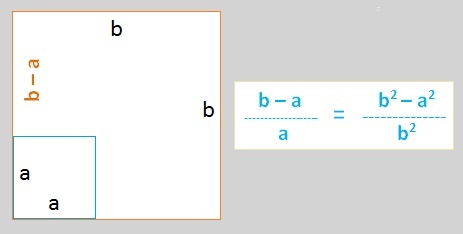# Fibonacci-like sequences and ratio of successive terms

Page 1   |  Page 2 of 3  |  Page 3

Before we try to find out the exact values, we note the following pattern. The golden ratio can be obtained using following geometric relation. Given a line segment, we divide the segment into 2 parts a and b, (b greater than a) such that b / a = (b + a) / b.     (Let's call this equation I)
This can be rearranged to give expression in the following image.To interpret this, consider a segment of length b. We divide the segment into 2 parts a and (b-a) such that ratio of (b - a) to smaller segment (a) = ratio of (area of square of side b - area of square of side a) to (area of square of side b). And this ratio is equal to the golden ratio 1.618..., which is also the value to which the order-2 sequence converges.
Question - Can we arrive at a similar geometric relation for the ratio of order-3 sequence, i.e, 1.839. Yes, we can! Similar to eqn. I, relation for 1.839...can be expressed as b / a = 1 + (a / b) + (a^2 / b^2).
This can be rearranged to the expression below:Here, we divide the segment into 2 parts a and b-a such that ratio of (b - a) to smaller segment (a) = ratio of (volume of cube of side b - volume of the cube of side a) to (volume of cube of side b). And this ratio is equal to the 1.839... (the value to which the order-3 sequence converges).
This pattern can be continued for higher order recurrence relations, where the geometric equivalent involves volumes of "hyper-cubes". Thus, in general, for sequence of order n,
(b - a) / a = ( b^n - a^n ) / b^n

Now, let's see how we arrive at the exact values of these ratios. I have not experimented with any closed from expression yet, but I was able to find out that all these ratios are roots of similar kind of equations, as we will see below. This I uncovered by generalizing the result for golden ratio (1.618...). From equation 1, let b/a = x. Then the equation becomes x = 1 + ( 1 / x ).
This can be simplified to    x^2 - x - 1 = 0.      One of the roots of this eqn is 1.618...
Thus, we generalize as below (and also prove later). Going back to the table on the previous page, we will add another column.

Number of
successive terms
Ratio     Root of following eqns. = Ratio
2 1.618...      x^2 - x - 1 = 0
3 1.839...      x^3 - x^2 - x - 1 = 0
4 1.928...      x^4 - x^3 - x^2 - x - 1 = 0
5 1.961...     x^5 - x^4 - x^3 - x^2 - x - 1 = 0
... ... .....

Thus, we started with a simple Fibonacci sequence, and discovered a few things (albeit trivial by contemporary mathematical standards) by asking simple follow-up questions. The next page will try to prove the claim that the ratios themselves converge to 2. If the topic still interests you, then feel free to continue reading here.

Page 1   |  Page 2 of 3  |  Page 3
 Quick Comment : * Name Email ( Your email id is safe with us! ) * Comments * Type the number of characters in the word "HUMAN"